Difference between revisions of "0708-1300/Homework Assignment 5"

Announcements go here

Contents

Read sections 1-3 of chapter V of Bredon's book three times:

• First time as if you were reading a novel - quickly and without too much attention to detail, just to learn what the main keywords and concepts and goals are.
• Second time like you were studying for an exam on the subject - slowly and not skipping anything, verifying every little detail.
• And then a third time, again at a quicker pace, to remind yourself of the bigger picture all those little details are there to paint.

Also, do the same with your own class notes - much of what we do for this part of the class is not in the textbook!

Doing

Solve all of the following problems, but submit only your solutions of problems 1, 3, 8, 9 and 10:

Problem 1. Let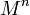$M^n$ be a manifold. Show that the following definitions for the orientability of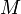$M$ are equivalent:

1. There exists a nowhere vanishing$n$-form on$M$.
2. There exists an atlas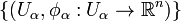$\{(U_\alpha,\phi_\alpha:U_\alpha\to{\mathbb R}^n)\}$ for$M$, so that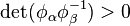$\det(\phi_\alpha\phi^{-1}_\beta)>0$ wherever that makes sense.

Problem 2. Show that the tangent space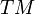$TM$ of any manifold$M$ is orientable.

Problem 3.

1. Show that if$M$ and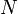$N$ are orientable then so is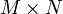$M\times N$.
2. Show that if$M$ and$M\times N$ are orientable then so is$N$.

Problem 4. Show that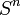$S^n$ is always orientable.

Problem 5. Recall that a form is called closed if it is in the kernel of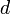$d$ and exact if it is in the image of$d$. Show that every exact form is closed.

Problem 6. Let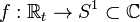$f:{\mathbb R}_t\to S^1\subset{\mathbb C}$ be given by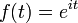$f(t)=e^{it}$.

1. Show that there exists a unique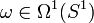$\omega\in\Omega^1(S^1)$ such that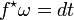$f^\star\omega=dt$.
2. Show that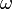$\omega$ is closed but not exact.

Problem 7. Show, directly from the definitions, that every closed 1-form on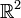${\mathbb R}^2$ is exact.

Problem 8. Compute the integral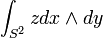$\int_{S^2}zdx\wedge dy$ twice:

1. Using Stokes' theorem.
2. Directly from the definition, by using a two- or three-chart atlas for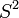$S^2$ (or for$S^2$ minus a single point).

(Repeat 1 and 2 until they stop giving different answers).

Problem 9. Show that the form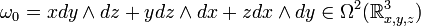$\omega_0=xdy\wedge dz+ydz\wedge dx+zdx\wedge dy\in\Omega^2({\mathbb R}^3_{x,y,z})$ is invariant under rigid orientation-preserving rotations of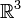${\mathbb R}^3$. That is, if$A$ is such a rotation matrix (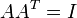$AA^T=I$ and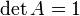$\det A=1$) considered also as a linear transformation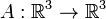$A:{\mathbb R}^3\to{\mathbb R}^3$, then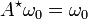$A^\star\omega_0=\omega_0$.

Problem 10. Let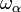$\omega_\alpha$ be the form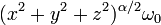$(x^2+y^2+z^2)^{\alpha/2}\omega_0$ defined on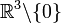${\mathbb R}^3\backslash\{0\}$, where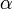$\alpha$ is a real number and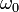$\omega_0$ is the form from the previous question.

1. Find a number$\alpha$ so that$\omega_\alpha$ would be closed.
2. For that value of$\alpha$, compute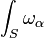$\int_S\omega_\alpha$, where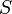$S$ is the sphere of radius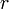$r$ around a point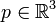$p\in{\mathbb R}^3$. (If you end up writing complicated formulas, you missed the point).
3. For the same$\alpha$, is$\omega_\alpha$ exact?

Due Date

This assignment is due in class on Thursday December 6, 2007.

Douglas Adams - "I love deadlines. I like the whooshing sound they make as they fly by."

Just for Fun

A planimeter is a (mechanical!) measuring instrument used to measure the area of an arbitrary two-dimensional shape by tracing along its boundary; if you have a map of Ontario, for example, you can roll the measuring end of a planimeter counterclockwise around the borders of the province and the gadget will tell you its area. Figure out how a planimeter works, both on the mechanical level and on the mathematical level. Wikipedia has an explanation which is is right in the spirit but wrong on the details. Fix it, if you feel inspired (and if you do right and let me know about it, it'll count as a good deed).